# Scaling US Census Income Category Prediction with Apache Spark (PySpark)¶

Have you ever wondered how Machine Learning Pipelines are built at scale? How can we deploy our ML models over a distributed cluster of machines so that our ML application scale horizontally? Do you want to convert your scikit-learn and pandas based ML workflow and take it to scale using Spark? Would it have been better if you had an working end-to-end application to refer? Then Read On...

@author: Anindya Saha @email: [email protected]

I hope you enjoy reviewing it as much as I had writing it.

## Why we use Apache Spark for ML?¶

Almost all of us who starts with Machine Learning in Python starts developing and learning using scikit-learn, pandas in Python. The datasets that we use fit in our laptops and can run with 16GB of memory. However, in reality when we go to the industry the datasets are not tiny and we need to scale with GBs of data over several machines. In order to scale our algorithm on large datasets scikit-learn, pandas are not enough. Spark is inherently designed for distributed computation and they have a MLlib package which has scalable implementation of most common algorithms.

Data Scientists usually work on a sample of the data to devise and tune their algorithms and when they are satisfied with the model's performance they then need to deploy that at scale in production. Sometimes they themselves do it or if you are working as an Applied ML Engineer or ML Software Engineer then the Data Scientist might seek your help in transforming his pandas, scikit-learn based codes into a more scalable and distributable deployment working over large datasets. While doing that soon you will realize not exactly everything of scikit-learn, pandas is implemented in Spark. Spark community is adding more ML implementations. But there are some constraints imposed by the distributed nature of the large data sets across machines that all features and functions that the Data Scientist have leveraged from Pandas is not available. Also, sometimes some functionalities such as StratifiedSampling will not be directly implemented in Spark. You have to use the existing Spark APIs to realize the same.

This post was inspired when a Graduate Data Science engineer sought my help to convert his own scikit-learn and pandas code to PySpark. While doing that I added more functionalities and implementation that you would find useful and handy and will serve as a ready reference implementation.

Although Spark is written in Python there is no inherent visualization capabilities. Here we will heavily leverage the toPandas() method to convert the aggregated DataFrames or results into Pandas DataFrame for visualization. This work covers - EDA, custom udf, cleaning, basic missing values treatment, data variance per feature, stratified sampling (custom implementation), class weights for imbalanced class distribution (custom code), onehotencoding, standard scaling, vector assembling, label encoding, grid search with cross validation, pipeline, partial pipelines (custom code), binary and multi class evaluators and new metrics introduced in Spark 2.3.0, auc_roc, roc curves, model serialization and deserialization.

## What is Apache Spark?¶Apache Spark is a fast cluster computing framework which is used for processing, querying and analyzing Big data. It is based on In-memory computation, which is a big advantage of Apache Spark over several other big data Frameworks. Apache Spark is open source and one of the most famous Big data framework. It can run tasks up to 100 times faster, when it utilizes the in-memory computations and 10 times faster when it uses disk than traditional map-reduce tasks. Read this article to learn more about Spark https://www.analyticsvidhya.com/blog/2016/09/comprehensive-introduction-to-apache-spark-rdds-dataframes-using-pyspark/

It provides high-level APIs in Java, Scala, Python and R, and an optimized engine that supports general execution graphs. It also supports a rich set of higher-level tools including Spark SQL for SQL and structured data processing, MLlib for machine learning, GraphX for graph processing, and Spark Streaming.

Even more: https://spark.apache.org/docs/latest/

In this notebook, we try to predict a person's income is above or below 50K$/yr based on features such as workclass, no of years of education, occupation, relationship, marital status, hours worked per week, race, sex etc. But the catch is here we will do entirely in PySpark. We will use the most basic model of Logistic Regression here. The goal of the notebook is not to get too fancy with the choice of the Algorithms but its more on how can you achieve or at least try to achieve what you could do using scikit-learn and pandas. ## BINARY CLASSIFICATION : LOGISTIC REGRESSION¶ US Adult Census data relating income to social factors such as Age, Education, race etc. The US Adult income dataset was extracted by Barry Becker from the 1994 US Census Database. The data set consists of anonymous information such as occupation, age, native country, race, capital gain, capital loss, education, work class and more. Each row is labelled as either having a salary greater than ">50K" or "<=50K". This Data set is split into two CSV files, named adult-training.txt and adult-test.txt. The goal here is to train a binary classifier on the training dataset to predict the column income_bracket which has two possible values ">50K" and "<=50K" and evaluate the accuracy of the classifier with the test dataset. Note that the dataset is made up of categorical and continuous features. It also contains missing values. The categorical columns are: workclass, education, marital_status, occupation, relationship, race, gender, native_country The continuous columns are: age, education_num, capital_gain, capital_loss, hours_per_week. This Dataset was obtained from the UCI repository, it can be found at: In : import os import pandas as pd import numpy as np from pyspark import SparkConf, SparkContext from pyspark.sql import SparkSession, SQLContext from pyspark.sql.types import * import pyspark.sql.functions as F from pyspark.sql.functions import col, udf from pyspark.ml.stat import Correlation from pyspark.ml.classification import LogisticRegression from pyspark.ml.tuning import ParamGridBuilder, CrossValidator, CrossValidatorModel from pyspark.ml.feature import Bucketizer, StringIndexer, OneHotEncoder, StandardScaler, VectorAssembler from pyspark.ml import Pipeline, PipelineModel from pyspark.ml.tuning import ParamGridBuilder, CrossValidator, CrossValidatorModel from pyspark.ml.evaluation import BinaryClassificationEvaluator from pyspark.mllib.evaluation import MulticlassMetrics  In : # Visualization import seaborn as sns import matplotlib.pyplot as plt  In : # Visualization from IPython.core.interactiveshell import InteractiveShell InteractiveShell.ast_node_interactivity = "all" pd.set_option('display.max_columns', 200) pd.set_option('display.max_colwidth', 400) sns.set(context='notebook', style='whitegrid', rc={"figure.figsize": (18,4)})  In : %matplotlib inline %config InlineBackend.figure_format = 'retina'  In : from matplotlib import rcParams rcParams['figure.figsize'] = 18,4  In : # setting random seed for notebook reproducability rnd_seed=42 np.random.seed=rnd_seed np.random.set_state=rnd_seed  ## 1. Creating the Spark Session¶ In : # The following must be set in your .bashrc file #SPARK_HOME="/home/ubuntu/spark-2.3.0-bin-hadoop2.7" #ANACONDA_HOME="/home/ubuntu/anaconda3/envs/pyspark" #PYSPARK_PYTHON="$ANACONDA_HOME/bin/python"
#PYSPARK_DRIVER_PYTHON="$ANACONDA_HOME/bin/python" #PYTHONPATH="$ANACONDA_HOME/bin/python"
#export PATH="$ANACONDA_HOME/bin:$SPARK_HOME/bin:\$PATH"

In :
spark = (SparkSession
.builder
.master("local[*]")
.appName("predict-us-census-income-python")
.getOrCreate())
spark

Out:

SparkSession - in-memory

SparkContext

Spark UI

Version
v2.3.0
Master
local[*]
AppName
predict-us-census-income
In :
sc = spark.sparkContext
sc

Out:

SparkContext

Spark UI

Version
v2.3.0
Master
local[*]
AppName
predict-us-census-income
In :
sqlContext = SQLContext(spark.sparkContext)
sqlContext

Out:
<pyspark.sql.context.SQLContext at 0x7fe06ab7a0f0>

## 2. Load The Data From a File Into a Dataframe¶

In :
ADULT_TRAIN_DATA = 'data/adult-training.csv'

In :
# define the schema, corresponding to a line in the csv data file.
schema = StructType([
StructField("age", IntegerType(), nullable=True),
StructField("workclass", StringType(), nullable=True),
StructField("fnlgwt", DoubleType(), nullable=True),
StructField("education", StringType(), nullable=True),
StructField("education_num", DoubleType(), nullable=True),
StructField("marital_status", StringType(), nullable=True),
StructField("occupation", StringType(), nullable=True),
StructField("relationship", StringType(), nullable=True),
StructField("race", StringType(), nullable=True),
StructField("sex", StringType(), nullable=True),
StructField("capital_gain", DoubleType(), nullable=True),
StructField("capital_loss", DoubleType(), nullable=True),
StructField("hours_per_week", DoubleType(), nullable=True),
StructField("native_country", StringType(), nullable=True),
StructField("income", StringType(), nullable=True)]
)

In :
# Load training data and cache it. We will be using this data set over and over again.
.cache())

In :
train_df.printSchema()

root
|-- age: integer (nullable = true)
|-- workclass: string (nullable = true)
|-- fnlgwt: double (nullable = true)
|-- education: string (nullable = true)
|-- education_num: double (nullable = true)
|-- marital_status: string (nullable = true)
|-- occupation: string (nullable = true)
|-- relationship: string (nullable = true)
|-- race: string (nullable = true)
|-- sex: string (nullable = true)
|-- capital_gain: double (nullable = true)
|-- capital_loss: double (nullable = true)
|-- hours_per_week: double (nullable = true)
|-- native_country: string (nullable = true)
|-- income: string (nullable = true)


In :
train_df.limit(10).toPandas()

Out:
age workclass fnlgwt education education_num marital_status occupation relationship race sex capital_gain capital_loss hours_per_week native_country income
0 39 State-gov 77516.0 Bachelors 13.0 Never-married Adm-clerical Not-in-family White Male 2174.0 0.0 40.0 United-States <=50K
1 50 Self-emp-not-inc 83311.0 Bachelors 13.0 Married-civ-spouse Exec-managerial Husband White Male 0.0 0.0 13.0 United-States <=50K
2 38 Private 215646.0 HS-grad 9.0 Divorced Handlers-cleaners Not-in-family White Male 0.0 0.0 40.0 United-States <=50K
3 53 Private 234721.0 11th 7.0 Married-civ-spouse Handlers-cleaners Husband Black Male 0.0 0.0 40.0 United-States <=50K
4 28 Private 338409.0 Bachelors 13.0 Married-civ-spouse Prof-specialty Wife Black Female 0.0 0.0 40.0 Cuba <=50K
5 37 Private 284582.0 Masters 14.0 Married-civ-spouse Exec-managerial Wife White Female 0.0 0.0 40.0 United-States <=50K
6 49 Private 160187.0 9th 5.0 Married-spouse-absent Other-service Not-in-family Black Female 0.0 0.0 16.0 Jamaica <=50K
7 52 Self-emp-not-inc 209642.0 HS-grad 9.0 Married-civ-spouse Exec-managerial Husband White Male 0.0 0.0 45.0 United-States >50K
8 31 Private 45781.0 Masters 14.0 Never-married Prof-specialty Not-in-family White Female 14084.0 0.0 50.0 United-States >50K
9 42 Private 159449.0 Bachelors 13.0 Married-civ-spouse Exec-managerial Husband White Male 5178.0 0.0 40.0 United-States >50K
In :
# Load testing data. We will be using this data set over and over again.
.cache())


## 3. Understanding the Distribution of various Features¶

Here we will do an EDA on the training set only. We will not be doing any EDA on the test data. It is supposed to be unknown data altogether. EDA should be limited to find out what type of cleaning is needed on test data. If it throws surprises in the end while testing, then we can go and do a thorough EDA to see if it was too off from training data.

3.1 How many records in each data set:

In :
print('Training Samples: {0}, Test Samples: {1}.'.format(train_df.count(), test_df.count()))

Training Samples: 32561, Test Samples: 16281.


3.2 What is the distribution of class labels in each data set:

In :
train_df.groupBy('income').count().withColumn('%age', F.round(col('count') / train_df.count(), 2)).toPandas()

Out:
income count %age
0 <=50K 24720 0.76
1 >50K 7841 0.24
In :
test_df.groupBy('income').count().withColumn('%age', F.round(col('count') / test_df.count(), 2)).toPandas()

Out:
income count %age
0 <=50K. 12435 0.76
1 >50K. 3846 0.24

We can clearly see a type in the income label for test set. The labels are <=50K. and >50K. instead of <=50K and >50K .

3.3 Fix Typos in Test Set:

In :
# There is a typo in the test set the values are '<=50K.' & '>50K.' instead of '<=50K' & '>50K'
test_df = test_df.replace(to_replace='<=50K.', value='<=50K', subset=['income'])
test_df = test_df.replace(to_replace='>50K.', value='>50K', subset=['income'])


3.4 How are the income labels distributed between the training set and the test set:

In :
fig, axes = plt.subplots(nrows=1, ncols=2, figsize=(10,4), sharex=False, sharey=False)

g = sns.countplot('income', data=train_df.select('income').toPandas(), ax=axes)
axes.set_title('Distribution of Income Groups in Test Set')

g = sns.countplot('income', data=test_df.select('income').toPandas(), ax=axes)
axes.set_title('Distribution of Income Groups in Test Set');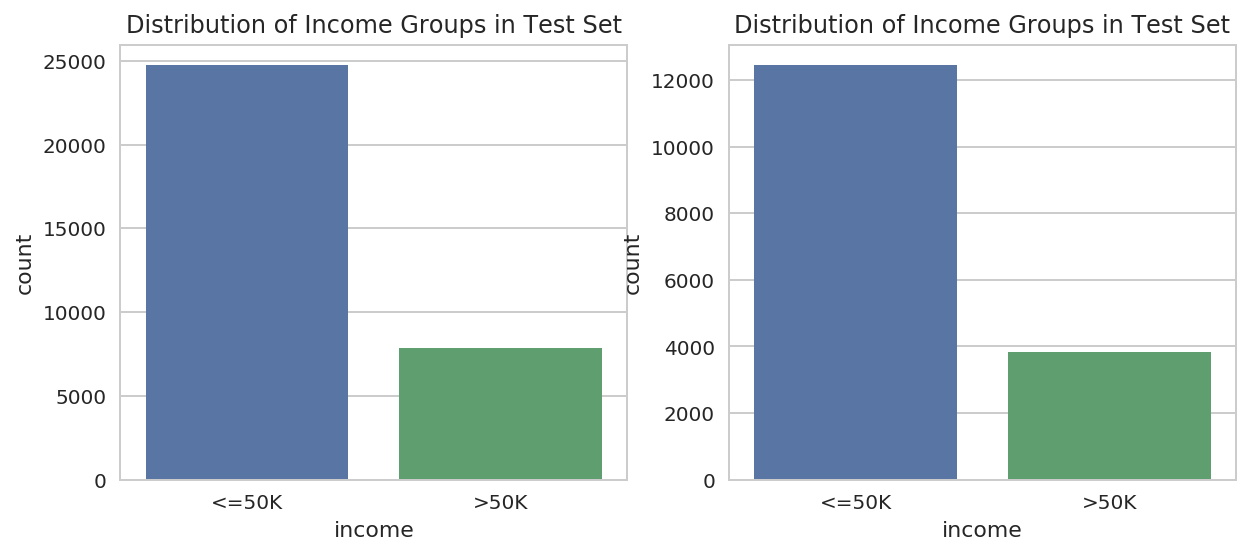We see almost the same distribution of income labels in the different data sets.

3.5 How is the Age distributed in the training set:

In :
sns.distplot(train_df.select('age').toPandas(), bins=100, color='red')
plt.title('Distribution of Age in Traning Set');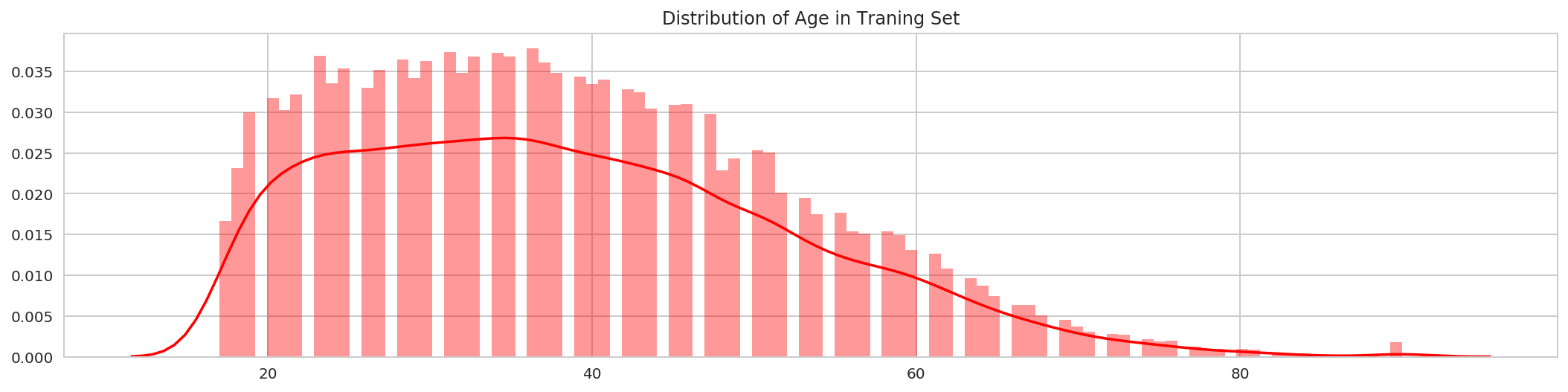Clearly, the age feature in right skewed and we can see some people working beyond the age of 80. Taking a logarithm of the age feature may be beneficial in turning this skewed distribution into a normal distribution.

Skewness of the Age Feature:

Skewness is a measure of the asymmetry of the probability distribution of a real-valued random variable about its mean. The skewness value can be positive or negative, or undefined. For a unimodal distribution, negative skew indicates that the tail on the left side of the probability density function is longer or fatter than the right side - it does not distinguish these two kinds of shape. Conversely, positive skew indicates that the tail on the right side is longer or fatter than the left side. https://en.wikipedia.org/wiki/Skewness

We can measure the skewness of a feature using Spark's skewness method. A variable is known to be moderately skewed if its absolute value is greater than 0.5 and highly skewed if its absolute value is greater than 1.

In :
# How skewed is the 'age' feature
train_df.select(F.skewness('age').alias('age_skew')).first()

Out:
Row(age_skew=0.5587176292398546)

Taking Logarithm Age Feature:

In :
sns.distplot(train_df.select(F.log10('age').alias('age')).toPandas(), bins=100, color='red')
plt.title('Distribution of Age in Traning Set');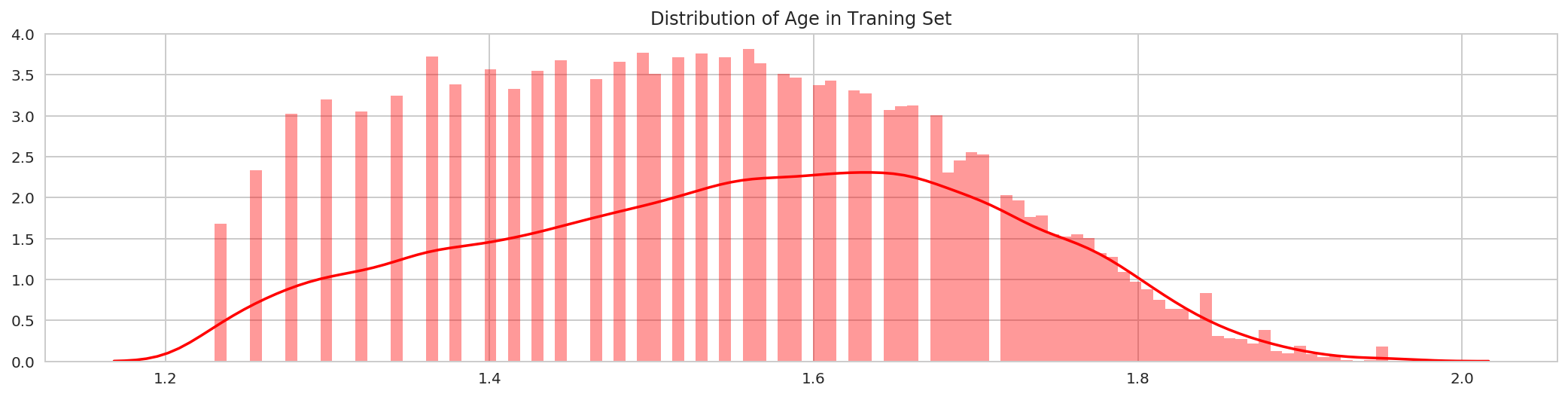In :
# How skewed is the 'log(age)' feature
train_df.select(F.skewness(F.log10('age')).alias('log_age_skew')).first()

Out:
Row(log_age_skew=-0.13172385088975794)

Taking the logarithm of the age feature reduces the skewness from moderate 0.55 to very low -0.13.

Some people who are working are beyond the age of 80. Are they self-employed or private? Are they earning <=50K?

In :
train_df.filter(col('age') > 80).select(['age', 'workclass', 'education', 'occupation', 'sex', 'income']).toPandas().head(10)

Out:
age workclass education occupation sex income
0 90 Private HS-grad Other-service Male <=50K
1 81 Self-emp-not-inc HS-grad Exec-managerial Male <=50K
2 90 Private HS-grad Other-service Female <=50K
3 88 Self-emp-not-inc Prof-school Prof-specialty Male <=50K
4 90 Private Bachelors Exec-managerial Male <=50K
5 90 Private Some-college Other-service Male <=50K
6 90 Private Some-college Adm-clerical Female <=50K
7 81 Private 9th Priv-house-serv Female <=50K
8 82 ? 7th-8th ? Male <=50K

Well, indeed the old people certainly earn <=50K but we cannot for sure say anything about the workclass or occupation.

In :
(train_df
.filter(col('age') > 80)
.groupby(['workclass', 'occupation'])
.count()
.orderBy(['workclass', 'occupation'])
.limit(10)
.toPandas())

Out:
workclass occupation count
0 ? ? 22
1 Federal-gov Craft-repair 1
3 Local-gov Exec-managerial 2
4 Local-gov Other-service 1
5 Local-gov Protective-serv 1
7 Private Craft-repair 2
8 Private Exec-managerial 9
9 Private Handlers-cleaners 2

There is one person working beyond the age of 80 in Federal Gov jobs.

3.6 How is the Hours Per Week distributed in the training set:

Hypothesis: We expect it to be more centered around 40 hours per week with occassional overtimes.

In :
sns.distplot(train_df.select('hours_per_week').toPandas(), bins=100, color='blue')
plt.title('Distribution of Hours Per Week in Traning Set');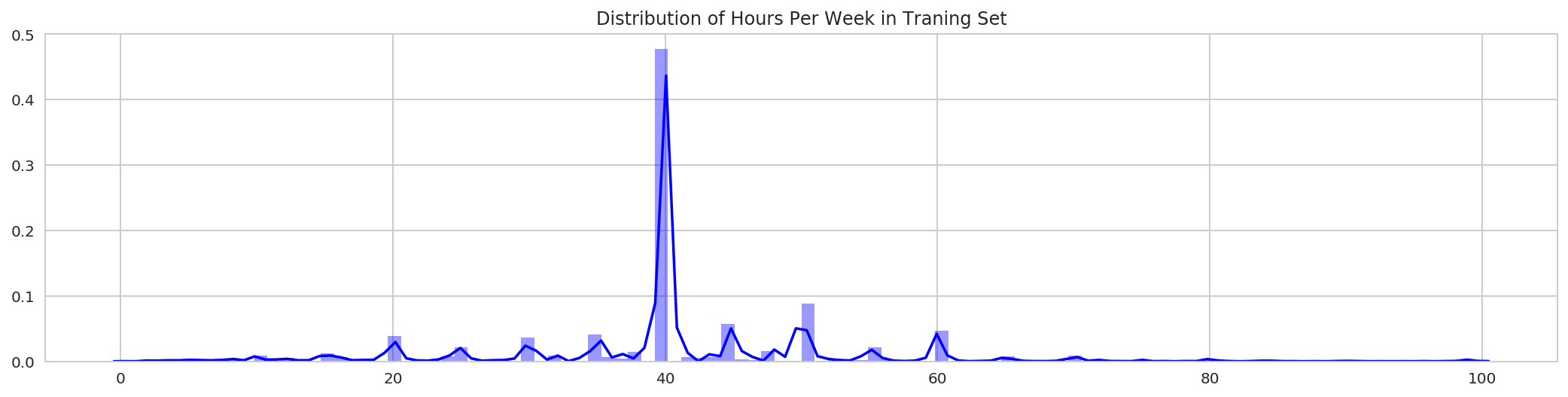It is indeed concentrated on 40 hours per week.

3.7 How is the Education_Num distributed in the training set:

In :
sns.distplot(train_df.select('education_num').toPandas(), bins=100, color='green')
plt.title('Distribution of Education Num in Traning Set');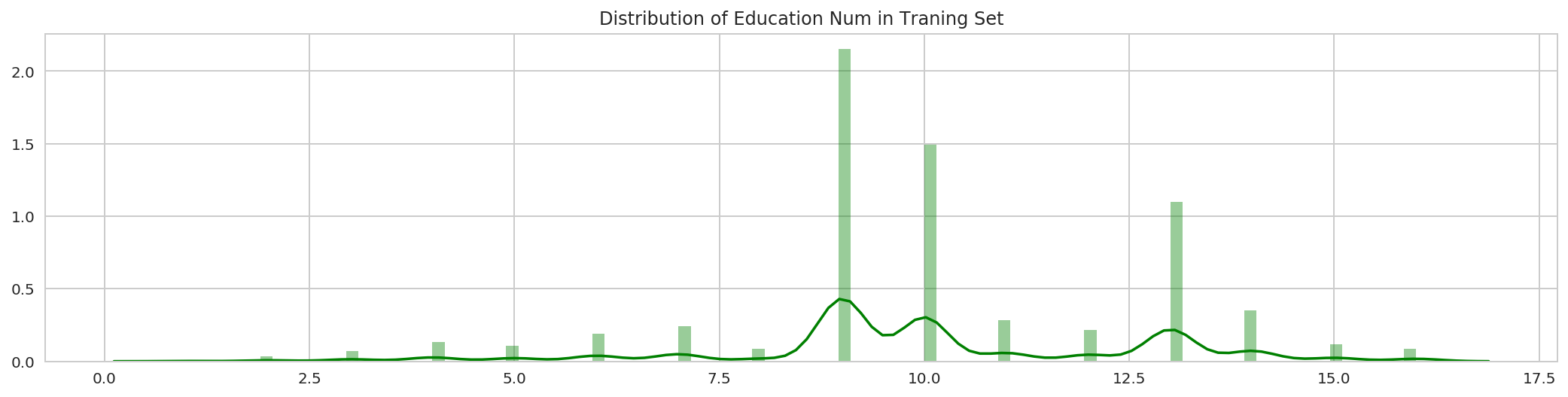3.8 How are the Capital Gain and Capital Loss attributes distributed in the training set:

In :
sns.distplot(train_df.select('capital_gain').toPandas(), bins=100, color='red')
plt.title('Distribution of Capital Gain in Traning Set');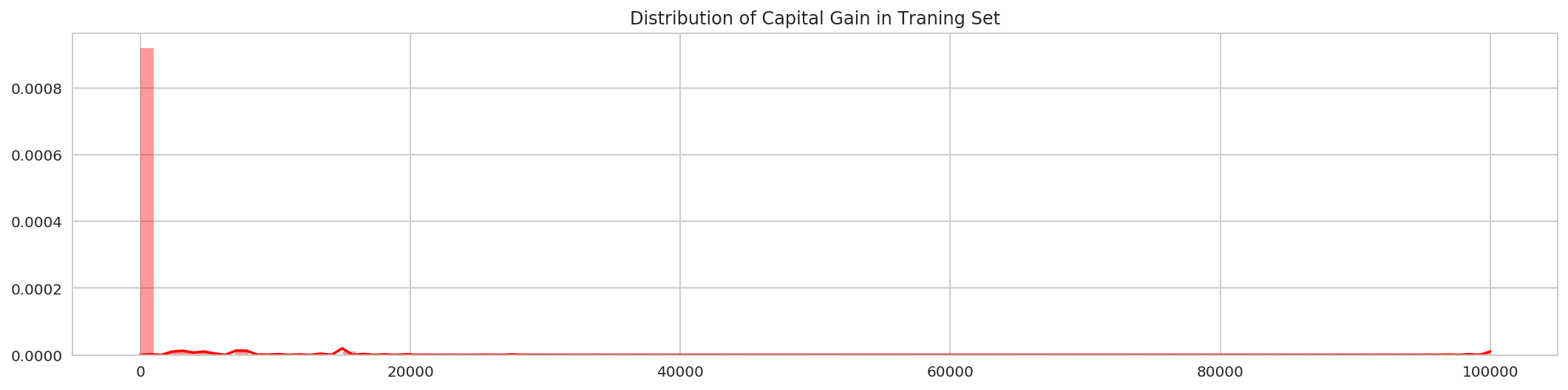In :
sns.distplot(train_df.select('capital_loss').toPandas(), bins=100, color='red')
plt.title('Distribution of Capital Loss in Traning Set');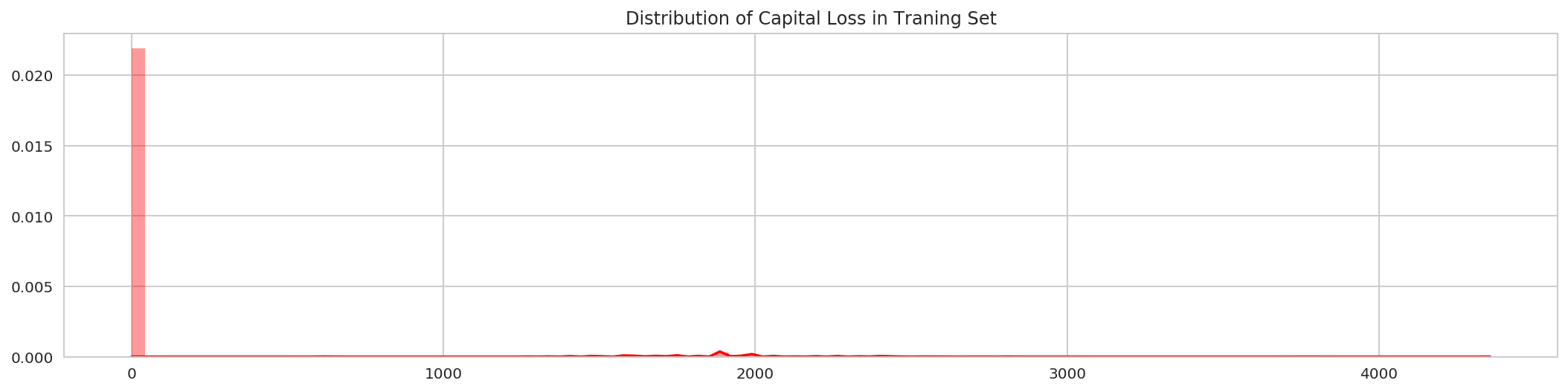The capital_gain and capital_loss are highly skewed. Let's check their skewness.

In :
# How skewed are the 'capital_gain' and 'capital_loss' feature
(train_df
.select(F.skewness('capital_gain').alias('capital_gain_skew'),
F.skewness('capital_loss').alias('capital_loss_skew'))
.first())

Out:
Row(capital_gain_skew=11.953296998194228, capital_loss_skew=4.594417456439665)

Following the graphs above the skewness value is very high for both of them are highly skewed. We will check with the correlation if they should be kept or dropped.

3.9 Correlation between the Numerical Attributes:

Let's investigate the correlation between the numerical features. Correlation measures whether there is any linear relationship between two features. Correlation measures whether increasing/decreasing a variable will also cause increase/decrease in the other variable. If two variables are highly correlated we can almost predict the one of the variable by looking at the values of the other variable. We calculate the Pearson correlation coefficient, which is sensitive only to a linear relationship between two variables. The Pearson correlation is +1 in the case of a perfect direct (increasing) linear relationship (correlation), −1 in the case of a perfect decreasing (inverse) linear relationship (anticorrelation), and some value in the open interval (−1, 1) in all other cases, indicating the degree of linear dependence between the variables. As it approaches zero there is less of a relationship (closer to uncorrelated). The closer the coefficient is to either −1 or 1, the stronger the correlation between the variables.

https://en.wikipedia.org/wiki/Correlation_and_dependence

Note: We cannot use DataFrame's corr method here because that only calculates the correlation of two columns of a DataFrame.

Note: We cannot calculate correlation for categorical features.

In :
# seggregate the numerical features
corr_columns = ['age', 'fnlgwt', 'education_num', 'capital_gain', 'capital_loss', 'hours_per_week']
train_df.select(corr_columns).limit(15).toPandas()

Out:
age fnlgwt education_num capital_gain capital_loss hours_per_week
0 39 77516.0 13.0 2174.0 0.0 40.0
1 50 83311.0 13.0 0.0 0.0 13.0
2 38 215646.0 9.0 0.0 0.0 40.0
3 53 234721.0 7.0 0.0 0.0 40.0
4 28 338409.0 13.0 0.0 0.0 40.0
5 37 284582.0 14.0 0.0 0.0 40.0
6 49 160187.0 5.0 0.0 0.0 16.0
7 52 209642.0 9.0 0.0 0.0 45.0
8 31 45781.0 14.0 14084.0 0.0 50.0
9 42 159449.0 13.0 5178.0 0.0 40.0
10 37 280464.0 10.0 0.0 0.0 80.0
11 30 141297.0 13.0 0.0 0.0 40.0
12 23 122272.0 13.0 0.0 0.0 30.0
13 32 205019.0 12.0 0.0 0.0 50.0
14 40 121772.0 11.0 0.0 0.0 40.0
In :
# Vectorize the numerical features first
corr_assembler = VectorAssembler(inputCols=corr_columns, outputCol="numerical_features")

# then apply the correlation package from stat module
pearsonCorr = (Correlation
.corr(corr_assembler.transform(train_df), column='numerical_features', method='pearson')
.collect())

In :
# convert to numpy array
pearsonCorr.toArray()

Out:
array([[  1.00000000e+00,  -7.66458679e-02,   3.65271895e-02,
7.76744982e-02,   5.77745395e-02,   6.87557075e-02],
[ -7.66458679e-02,   1.00000000e+00,  -4.31946327e-02,
4.31885792e-04,  -1.02517117e-02,  -1.87684906e-02],
[  3.65271895e-02,  -4.31946327e-02,   1.00000000e+00,
1.22630115e-01,   7.99229567e-02,   1.48122733e-01],
[  7.76744982e-02,   4.31885792e-04,   1.22630115e-01,
1.00000000e+00,  -3.16150630e-02,   7.84086154e-02],
[  5.77745395e-02,  -1.02517117e-02,   7.99229567e-02,
-3.16150630e-02,   1.00000000e+00,   5.42563623e-02],
[  6.87557075e-02,  -1.87684906e-02,   1.48122733e-01,
7.84086154e-02,   5.42563623e-02,   1.00000000e+00]])
In :
# Compute the correlation matrix
corrMatt = pearsonCorr.toArray()

# Generate a mask for the upper triangle

# Set up the matplotlib figure
plt.title('Numerical Features Correlation')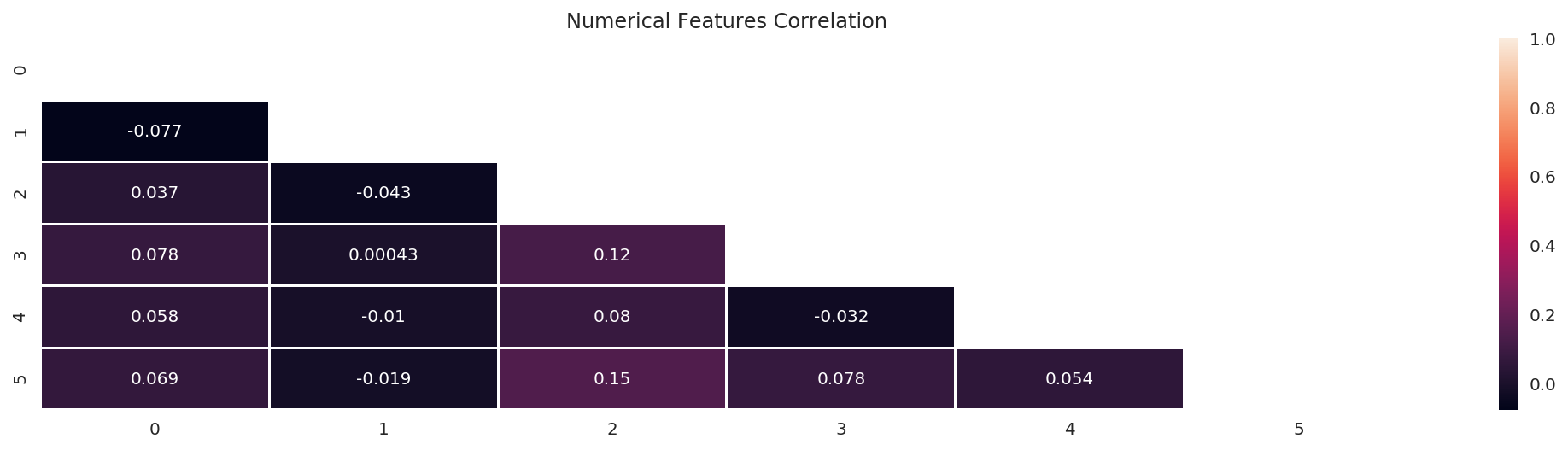It is clear from the heat map that none of the numerical features are correlated with each other.

3.10 How is the Income distributed across Workclass in the training set:

In :
sns.countplot(y='workclass', hue='income', data=train_df.select('workclass', 'income').toPandas(), palette="viridis")
plt.title('Distribution of income vs workclass in training set');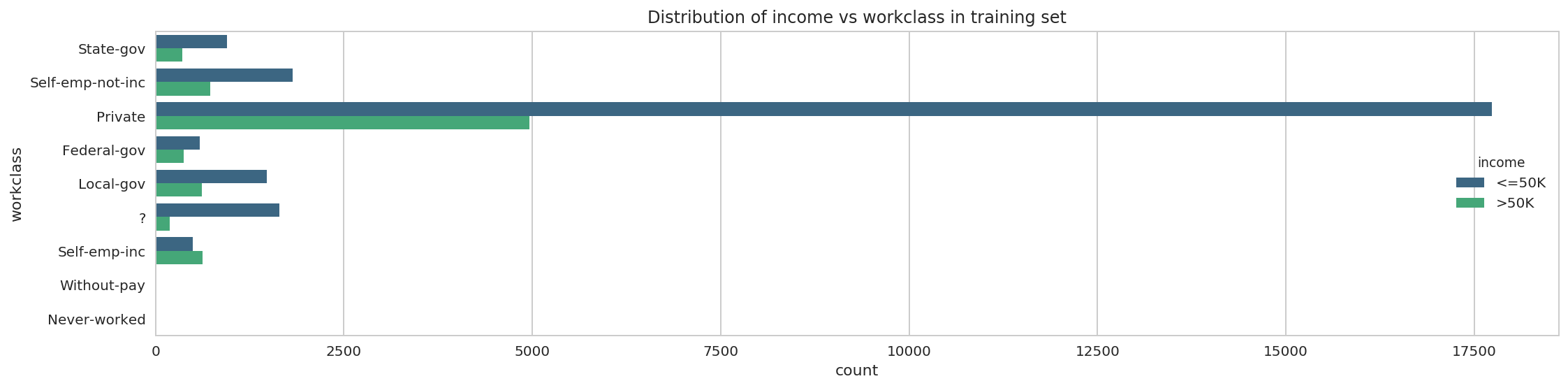Most people are employed in the Private Sector. Since, there are so many people working in private sector we can create two separate models, one for the private sector and one for the rest of the sectors.

Observing the unique values for the workclass columns we can see there are is a special character '?'. It most likely signifies NaN or unknown values and we have to handle it later.

3.11 How is the Income distributed across Occupation in the training set:

Does Intellectual Skill Sets / Higher Skill Sets / Specialized skill sets ensure Higher Income?

In :
sns.countplot(x='occupation', hue='income', data=train_df.select('occupation', 'income').toPandas(), palette="viridis")
plt.title('Distribution of income vs occupation in training set')
plt.xticks(rotation=45, ha='right');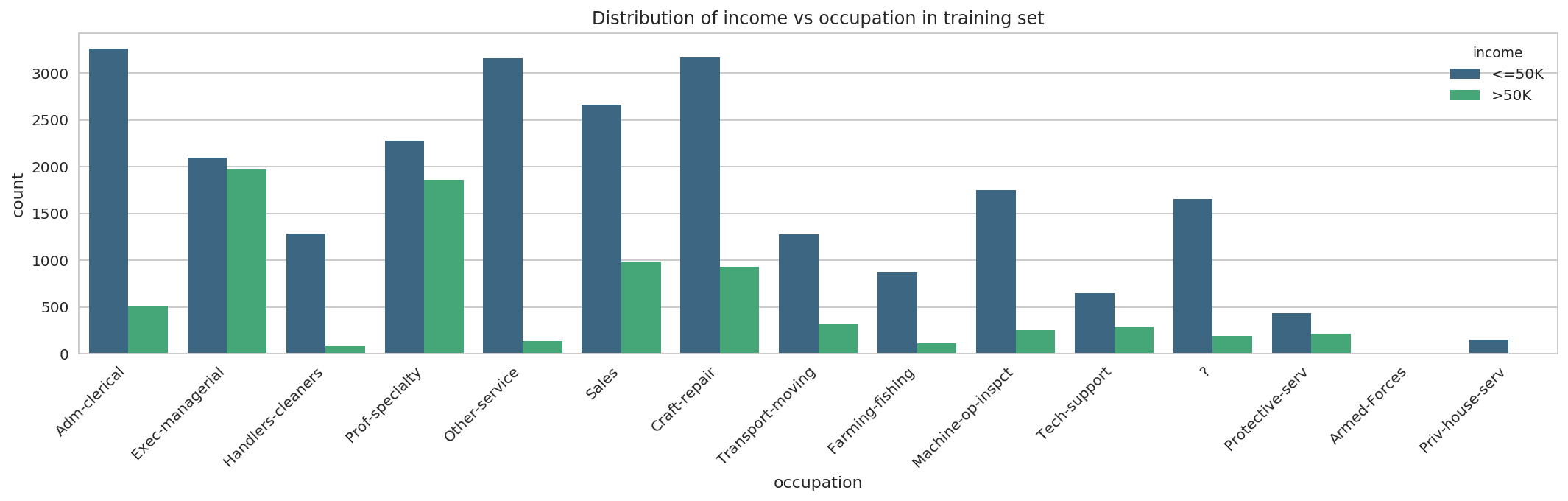Although, it is evident that the proportion of people earning >50K is higher in the specialized skillsets as compared to low skilled labor, but even within the high skilled specification it is not necessary that one will always have higher income as can be seen in the case of 'Exec-managerial' and 'Prof-speciality'.

3.12 How is the Income distributed across age and hours_per_week in the training set:

Hypothesis 1: For most people the prime time of their career is between 35-45 and that is where they are likely earn higher income.

Hypothesis 2: If we work hard and put in more hours per week to work we should earn more.

In :
fig, axes = plt.subplots(1, 2, figsize=(10,4), sharex=False, sharey=False)

sns.violinplot(x='income', y='age', data=train_df.select('age', 'income').toPandas(), ax=axes, palette="Set3")
axes.set_title('age vs income')

sns.violinplot(x='income', y='hours_per_week', data=train_df.select('hours_per_week', 'income').toPandas(), ax=axes, palette="Set2")
axes.set_title('hours_per_week vs income')

fig.suptitle('Training Set');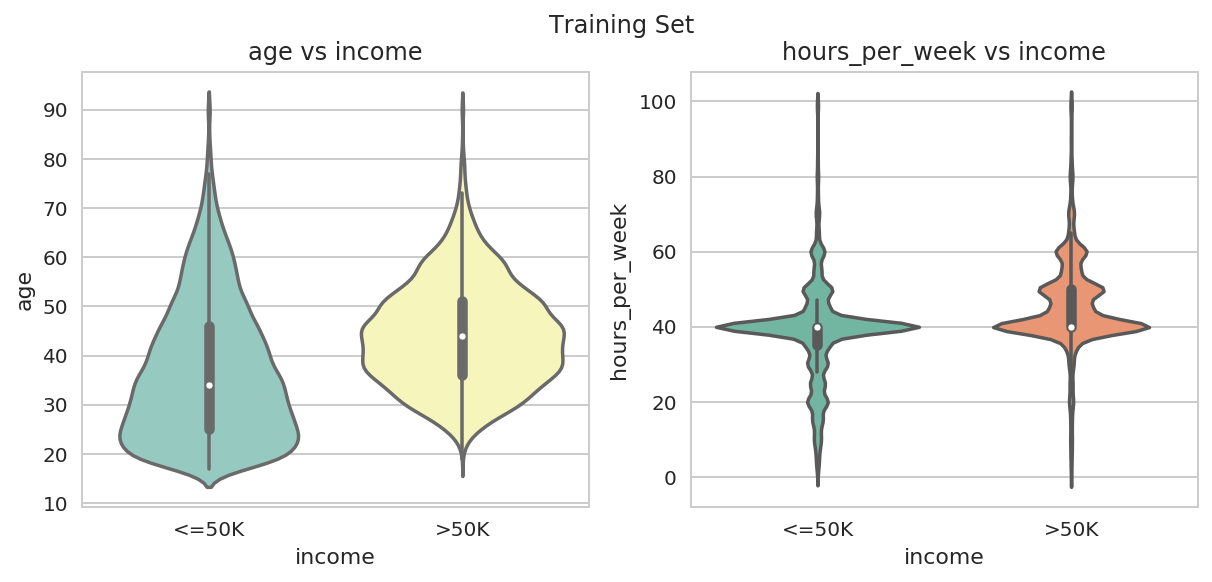Hypothesis 1: This hypothesis holds good from the first violin plot as we can see that the bulk of people who is earning >50K are in the age range 35-55. Our income is less during our older years or very younger years.

Hypothesis 2: This hypothesis is rejected because greater number of hours does not relate to higher income. There is same distribution of number of people across the hours in both earning categories of <50K or >=50K. Perhaps, higher income is determined by other factors such as education, experience etc.

3.13 How is the Income distributed across marital_status in the training set:

In :
sns.countplot(y='marital_status', hue='income', data=train_df.select('marital_status', 'income').toPandas())
plt.title('marital_status vs income in training set');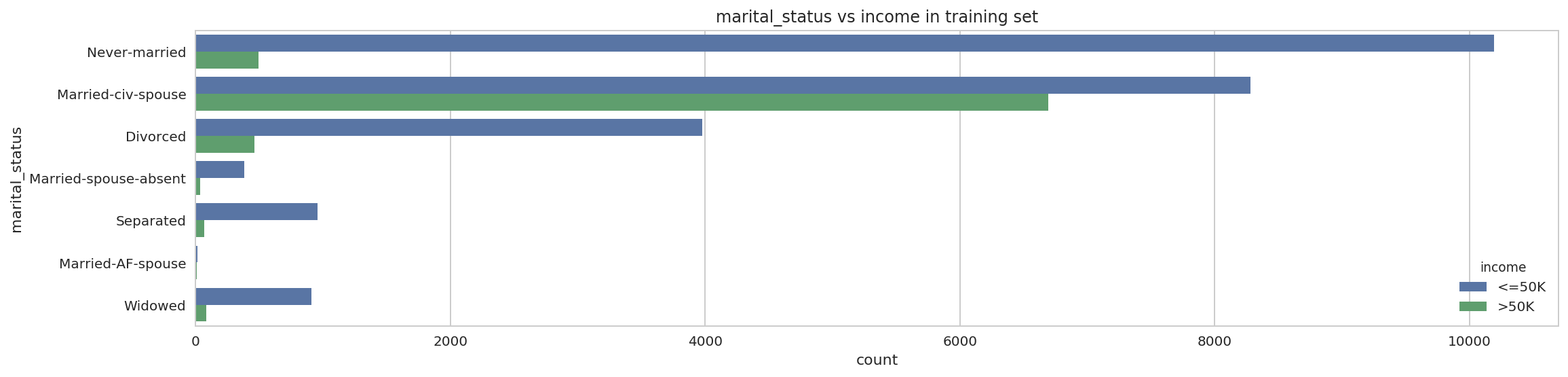Quite interestingly significant proportion of Married Couples have income >50K. Does it signify joint income?

3.14 How is the Income distributed across native_country and education_num in the training set:

How important is higher education for higher income?

In :
sns.violinplot(x="native_country", y="education_num", hue="income", data=train_df.select('native_country', 'education_num', 'income').toPandas(), inner="quartile", split=True)
plt.title('native_country and education_num across income level in training set')
plt.xticks(rotation=45, ha='right');